Steam Turbines

James M. Glownia October 31, 2007

(Submitted as coursework for Physics 210, Stanford University, Fall 2007)

In general, a turbine is a device in which a working fluid performs work on an element attached to a rotating shaft. Since steam has a relatively high latent heat, enormous thermodynamic potential energy can be stored in steam, and hence turbines often use steam as the working fluid. However, this thermal energy cannot be directly utilized and it must be first converted to kinetic energy through rapid expansion in a nozzle. This fast steam subsequently does work on the turbine blades, allowing useful power to be produced which explains why steam turbines to this day have a prominent place in power generation systems.

Thermodynamic Work and Enthalpy

Water is perhaps the most common working fluid in turbines with good reason. Water is cheap, abundant, has a reasonable boiling point, has intermediate density, and most importantly has a relatively high latent heat. The definition of latent heat, or enthalpy, is h = u - p V, where u is the total thermodynamic potential energy, p is pressure, and V is volume. Thus, the thermodynamic potential energy increases linearly with enthalpy. However, this offers little insight into how this energy can be used to perform useful work. To better understand this, we must look directly at the first law of engineering thermodynamics as applied to a control volume with mass flow m, enthalpy h, velocity v, and physical height z evaluated at the ends of the control volume: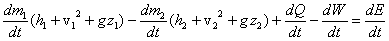This is simply a conservation of energy relation where h is the thermodynamic potential energy, v2 is the kinetic energy, gz is the gravity potential energy, Q is the system heat input, W is work done by the system, and E is the total energy lost by the system. For a mass conserving system and assuming well behaved variables we can write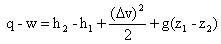If we can assume that the turbine is well insulated (which is normally the case) along with the fact that the gravity potential energy does not change much (a reasonable assumption for small turbines), it is clear that the work produced by the system is directly related to the change in enthalpy as well as the change in kinetic energy of the system. Since the kinetic energy of the superheated steam passing into the turbine, as well as the low pressure steam passing out of the turbine, is orders of magnitude less than the change in enthalpy, the change in kinetic energy of the system is also not significant. Thus, the amount of available work from the turbine, barring frictional losses, is simply the change in enthalpy from the superheated steam to the low pressure steam output by the turbine, or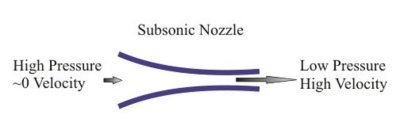Fig. 1: Typical Nozzle Geometry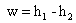Nozzles

The expansion of gas through a nozzle (Fig. 1) is an example of a reversible process in which approximately no heat is lost and no work is performed. This is an example of an isentropic process wherein the entropy of the system remains constant. We utilize this property through the thermodynamic relation T ds = dh - V dp to solve for the fluid velocities exiting a nozzle. Setting the above equation to zero and substituting the result into the appropriate first law expression, we get dv = -dh/v. This result, in conjunction with the fluid continuity equation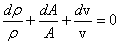yields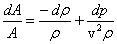Since the speed of sound is c = (d p/d ρ)½, with the Mach number (M) defined as v/c, using the Euler equation gives the following result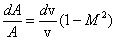It now evident that in the case for subsonic flow, when dA/A decreases, as occurs for restricted flow, dv/v must correspondingly increase, as expected. Also, as the output velocity becomes supersonic, the flow becomes choked. In this choked flow regime, the nozzle actually acts as a diffuser, which decreases rather than increases the steam's velocity, which is undesirable. Hence special supersonic nozzles are designed with diverging face orifices to help minimize choked flow.

We can also use Euler equation to solve for the change in enthalpy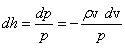Here it is explicit that the flow of steam through a nozzle serves to increase its velocity, but this is at the expense of enthalpy, which is a key concept behind steam turbine operation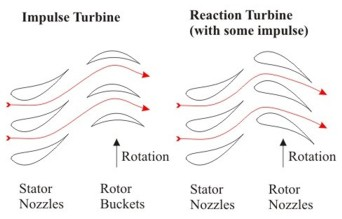Fig. 2: Side view of two turbine types showing example steam flow lines. The stationary blades are arranged to form nozzles in both examples. The rotor buckets in the impulse turbine act like paddle wheels while the rotor blades in the reaction turbine are actually rotating nozzles that are similar to those found in the stator nozzles.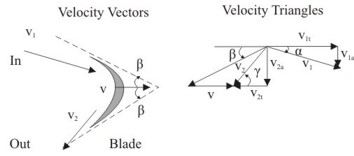Fig. 3: An example velocity diagram with the corresponding component vectors.

Turbine Mechanics

Turbines utilize nozzles (area restrictions) to increase the kinetic energy of the input steam. The steam is then allowed to collide with axial mounted turbine blades (also called buckets) to harness steam's kinetic energy and produce useful torque. There are two general types of turbine construction that contain either stationary nozzles or rotating nozzles (Fig. 2), and the general analysis of these turbines will be treated independently.

Impulse Turbines

Impulse Turbines contain stationary nozzles that direct steam onto rotating blades. We start with the enthalpy energy equation, which if analyzed in terms of the integrated kinetic energy expression from the last section, is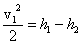implying that the equation governing the nozzle exit velocity (v1) is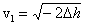The kinetic steam impinging upon a turbine blade, redirected as show in Fig. 3, results in a net tangential force F on the turbine blade. Using the usual laws governing force and applying vector algebra, an expression for the power generated (ignoring friction) is:Thus, the power transferred to the turbine is simply related to the difference of the input and output steam kinetic energies. This result highlights the point that although the might of steam lies in its stored thermodynamic energy, the actual power generated in steam turbines derives from pure kinetic energy.

Reaction Turbines

Reaction Turbines contain rotating nozzles and are similar in principle to rotary lawn sprinklers where the reaction force from a fluid produces torque. In steam turbines it is steam exiting nozzles that produces torque, where one of the earliest known steam turbines, described by Hero around 100 BC , was a reaction turbine. However, since such turbines are relatively primitive and not very efficient, almost all turbines in operation today are a combination of reaction and impulse turbines. These turbines are very complex to analyze and only a small amount analysis for an ideal case will be presented here.

To start the analysis we use our prior result for reaction turbines where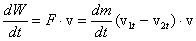.

However, to simply matters, we make several ideal case approximations in order to obtain a compact result. The first assumption is that v2t is zero, which, in some cases may be a reasonable assumption, but in practice, is nearly impossible to implement. Also, when considering the vector geometry of the blade, it is clear that the ideal blade velocity speed for maximum power is v = v1cos(α) Thus we can obtain an estimate for the maximum power from a reaction turbine:.

This ideal result simplifies to the reaction turbine case for the maximum efficiency where the input angle (alpha) equals zero. Since this is not practical in reality the extra loss can be overcome by allowing the turbine to be part reaction and impulse. Here again the power provided to the turbine is provided directly by the kinetic energy of expanded steam.

Staged Turbines

After considering this analysis it is perhaps natural to question where each turbine type is best used, and how efficiency can be increased. No single turbine stage can be very efficient due to flow restrictions, friction, condensation, and other factors. Naturally, there are engineering solutions to optimize performance where most turbines are staged meaning that the same steam serially flows through several different turbine assemblies, or stages, to increase torque and overall efficiency. An example of a stacked Rateau stage is shown in Fig. 4. As the steam passes through this assemble, its velocity increases in the nozzle, drops in the impulse turbine, remains constant through a set of stationary turning blades, and then drops again in the subsequent impulse rotary stage, as expected. It is also evident that the pressure drops in the nozzles and otherwise remains constant throughout the rest of the turbine, as these are free from area restrictions. This is advantageous since relatively high pressure steam exhausted by this stage can easily be reheated and further expanded in subsequent reaction type low pressure stages.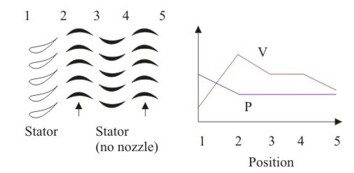Fig. 4: Staged impulse turbine showing the relative velocities and pressures after each stage. Note the relatively high pressure at the output.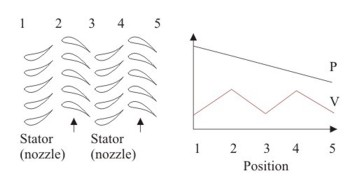Fig. 5: Staged reactive turbine showing the relative velocities and pressures after each stage.

An example of a reaction staged turbine is shown in Fig. 5. Sets of stationary nozzles are used to peak the input velocity for each reaction rotor stage, while each rotor itself is a nozzle that acts to reduce the absolute speed of the steam due to the work performed. Because the steam is expanded in each stage, the pressure drops consistently in each stage. Thus, the exhaust steam pressure from a reaction turbine is relatively low, and it is therefore advantageous to condense and compress the low pressure steam for re-use in the boiler.

Power Example

Steam turbines are used to produce over 86% of the electrical power in the world. They are used primarily because of their efficiency and their ability to generate enormous amounts of torque while containing relatively few separate moving parts. As a realistic example consider that a possible several hundred megawatt power plant boiler supplies steam at 20 MPa (20 atmospheres) and at 500 °C while the turbine outlet steam is at 4Mpa and at 300 °C. Using the steam tables and the general enthalpy relations above we find that an ideal turbine can create 2924.3 kJ per kg of steam which is an incredible amount of power. To put this in perspective one kJ of steam can generate the same amount of energy as the kinetic energy of a small car dropped off the top of the empire state building. A plant using this turbine could easily produce roughly 400-500 MW of electricity continuously.

© 2007 James M. Glownia. The author grants permission to copy, distribute and display this work in unaltered form, with attribution to the author, for noncommercial purposes only. All other rights, including commercial rights, are reserved to the author.

References

 R. Daugherty, J. Franzini, E. Finnemore, Fluid Mechanics with Engineering Applications (8th ed) (McGraw-Hill, 1985).

 D. Look and H. Sauer, Engineering Thermodynamics (PWS Engineering, 1986).

 L. Derr (editor), Cyclopedia of Engineering (American Technical Society, 1919).

 R. Turton, Principles of Turbomachinery (2nd ed) (Chapman and Hall 1995).

 Wikipedia contributors, "steam turbines," Wikipedia, The Free Encyclopedia, (accessed October 24, 2007).